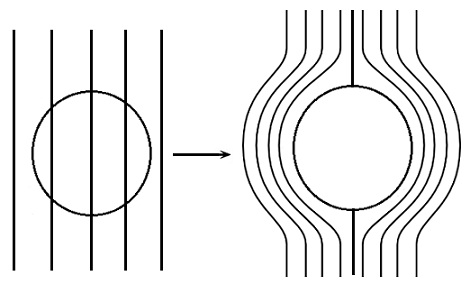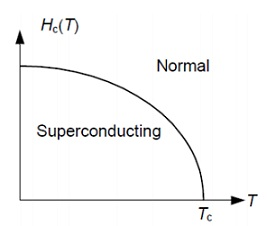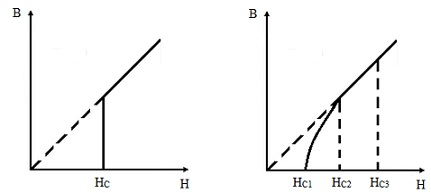#### Superconductivity-Experiments and Theories, Physics tutorial

Definition:

The Superconductivity is a phenomenon on which the electrical resistivity of metals or alloys drop to zero (that is, infinite conductivity) if cooled to its critical temperature.

Meissner effect:

Meissner and Ochsenfeld in the year 1933 illustrated that, if a long superconductor is cooled in a longitudinal magnetic field from above the transition temperature, the lines of induction are pushed out (figure shown below) at the transition. The Meissner effect illustrates that a super-conductor behaves as if within the specimen B = 0 or χ = - 1/4π; that is, a superconductor shows perfect diamagnetism. This very significant result can't be derived merely from the characterization of a superconductor as the medium of zero resistivity ρ: from E = ρj we observe that, if ρ is zero whereas j is finite, then E should be zero and with it curl E should be zero. Thus from the Maxwell's equations:

dB/dt = - curl E = 0

So that the flux via the metal can't change on cooling via the transition. The Meissner effect challenges this result and proposes that perfect diamagnetism and zero resistivity are two independent necessary properties of the superconducting state.Critical Field:

The minimum applied magnetic field essential to destroy the superconductivity and restore the normal resistivity is termed as the critical field, HC. HC depends on the temperature. The figure shown below represents the critical field as the function of temperature. The curve is almost parabolic and can be reasonably well symbolized by the relation:

HC = Ho [1 - (T/TC)2]

Here, Ho is the critical field at absolute zero. This equation is in reality the equation of phase boundary between the normal and superconducting state. The usual value of Ho is 5000A/m.Type I and Type II Superconductors:

Superconductors might be categorized into two classes that depend on the manner in which the transition from the superconducting to the normal state proceeds if the applied field exceeds HC. In type-I materials, as HC is reached the whole specimen enter the normal state practically concurrently, the resistance returns, the diamagnetic moment becomes zero and Binternal = Bexternal The figure is as shown below:In type-II superconductors, the transition to a wholly normal specimen is much steadier. As illustrated in the second part of the figure above, there is a partial penetration of the magnetic field between the critical field HC1 and HC2. Small surface super currents might still flow up to an applied field HC3.

Critical Currents:

The minimum current which can be passed in a sample devoid of destroying its superconductivity is termed as the critical current IC. If a wire (having radius r) of a type-I superconductor carries a current 'I', there is a surface magnetic field HI = I/2πr related by the current. Whenever HI exceeds HC, the material will go normal. If in addition, a transverse magnetic field 'H' is applied to the wire, the condition for the transition to the normal state at the surface is that the sum of the applied field and the field due to the current must equivalent the critical field. Therefore, as seen from the second part of the figure shown below, we have:

Ho = HI + 2H

HI = (Io/2πr) = Ho - 2H

Hence Io = 2πr (Ho - 2H)

The critical current IC will reduce linearly by the increase of the applied field until it reaches zero at H = HC/2. If the applied field is zero, IC = 2πrHC, like considerations apply to type-II superconductor for H < HC1, that is if the superconductor is not in the mixed state.London Equations:

In the year 1935, two brothers F. and H. London, introduced two equations to administer the microscopic electric and magnetic fields (that is, two fundamental electrodynamics properties) that provide superconductivity its unique interest. The London theory is mainly based on rather old ideas of the two-fluid model according to which a superconductor can be supposed to be comprised of both normal and super fluid electrons. Assume that nn, vn and ns, vs are correspondingly the density, and velocity of the normal and super fluid electrons. If no is the number of electrons per unit volume, then on the average:

no = nn + ns

The equation of motion for the super-fluid electrons is represented by:

m dvs/dt = - eE

The density of the super-fluid electrons is represented by:

js = - ensvs

Then, from the above two equation, we get:

djs/dt = (nse2/m) E

The above is the first London equation.

Taking curl of the above equation, we get:

∇ x (djs/dt) = (nse2/m) curl E

And by employing the Maxwell's equation, we get:

Curl E = - ∂B/∂t

We obtain, ∇ x (djs/dt) = - (nse2/m) ∂B/∂t

Integrating the above equation with respect to time, and selecting the constant of integration to be zero consistent by the Meissner effect, we obtain:

∇ x js = - (nse2/m) B

The above equation is the second London equation.

We might derive the Meissner effect from the second London equation by employing the Maxwell equation:

∇ x B = μojs

By taking the curl of the above equation, we get:

curl curl B = μo∇ x js

Then by using the condition div B = 0 of a superconductor in the identity:

curl curl B = grad div B - ∇2B

We obtain, curl curl B = - ∇2B

On combining the two above equation, we get:

2B = (1/λ2) B

Here 'λ' is termed as the London penetration depth and is stated by:

λ = (m/μonse2)1/2

For a superconductor to the right of the plane x = 0, the equation ∇2B = (1/λ2) B has the solution

B(x) = B(O) exp (- x/λ)

This equation points out that B doesn't penetrate much deeply into superconductor, and thus it means the Meissner effect. The field penetrates only a distance 'λ' in the surface. 'λ' is typically of the order of 1000Å. The graphical form of the equation is as shown in the figure given below. The penetration depth is as well found to depend strongly on temperature and to become much bigger as T approaches TC. The observation can be fitted extremely well through a simple expression of the form:

[λ(T)/λ(O)]2 = [1 - (T/TC)4]-1

This equation means that:

ns = ns [1 - (T/TC)4]-1The density superconducting electrons rise from zero at TC to no at absolute zero as illustrated in the figure shown below, which as well shows the temperature variation of λ . ns is termed as the order parameter as it characterizes the order in the superconducting state.Thermodynamics of Superconducting transition:

This has been illustrated experimentally that the transition between the normal and superconducting states is thermodynamically reversible, in the similar sense that with slow evaporation the transition between liquid and vapor phases of a substance is reversible. The Meissner effect as well recommends that the transition is reversible and would not subsist if the superconducting currents die away by the production of Joule heat if superconductivity is destroyed. As the transition is reversible we might apply thermodynamics to the transition, achieving an expression for the entropy difference between the normal and superconducting states in terms of the critical field curve HC versus TC.

The Gibbs free energy per unit volume in the magnetic field:

G = U - TS + PV - MH/μo

Then the differential Gibbs free energy dG is:

dG = - S dT + PdV - MdH/μo

At constant T and P, the free energy difference, due to the presence of a magnetic field, is found through integration. Therefore,

T,oT,H dG = - oH (M/μo) dH

G(T,H) - G(T,O) = - oH (M/μo) dH

For superconductor, I = - H or M = - VH and

GS (T,H) - GS (T,O) = - oH (VH/μo) dH

GS (T,H) - GS (T,O) = (1/2μo)(VH)2

In this, GS is the free energy of the superconducting state.

All along the phase boundary between normal and superconducting state, the normal phase should encompass a free energy indistinguishable from that of the superconducting phase. Thus,

Gn(T, HC) - GS(T, O) = (1/2μo)(VHC2)

Here, Gn is the free energy of the normal phase.

Let us now compute the difference in entropy of the two states. For solids, the entropy 'S' is represented by - dG/dT. Therefore, differentiating the above equation with respect to T, we obtain:

Sn - SS = - d/dT (1/2μo VHC2)

Sn - SS = - (VHCo)(dHC/dT)

Here, the entropies Sn and Sn refer to normal and superconducting states correspondingly.

The latent heat absorbed if the superconductivity is destroyed is:

Q = T(Sn - SS)

Q = - (VT/μo) HC (dHC/dT)

In the absence of a magnetic field, the transition takes place at TC and the latent heat is zero. If Un and US are correspondingly the normal and superconducting state internal energies, then from the above equation, we have:

Un - US = T(Sn - SS)

Un - US = - (VTHCo) (dHC/dT)

From the experiment, Sn - SS ≈ 10-7 eV that is extremely small as compared to the band energies. For a unit volume, the difference of the of the heat capacities, from the above equation, will be:

(CS - Cn) = T(d/dT) (SS - Sn)

(CS - Cn) = (THCo) (d2HC/dT2) + (T/μo) (dHC/dT)2

On replacing T = Tc, HC = 0 in this equation, we obtain the Rugers formula:

(CS - Cn) = (TCo)(dHC/dT)2 T=TC

The above equation repeats the experimental data very well.

Tutorsglobe: A way to secure high grade in your curriculum (Online Tutoring)